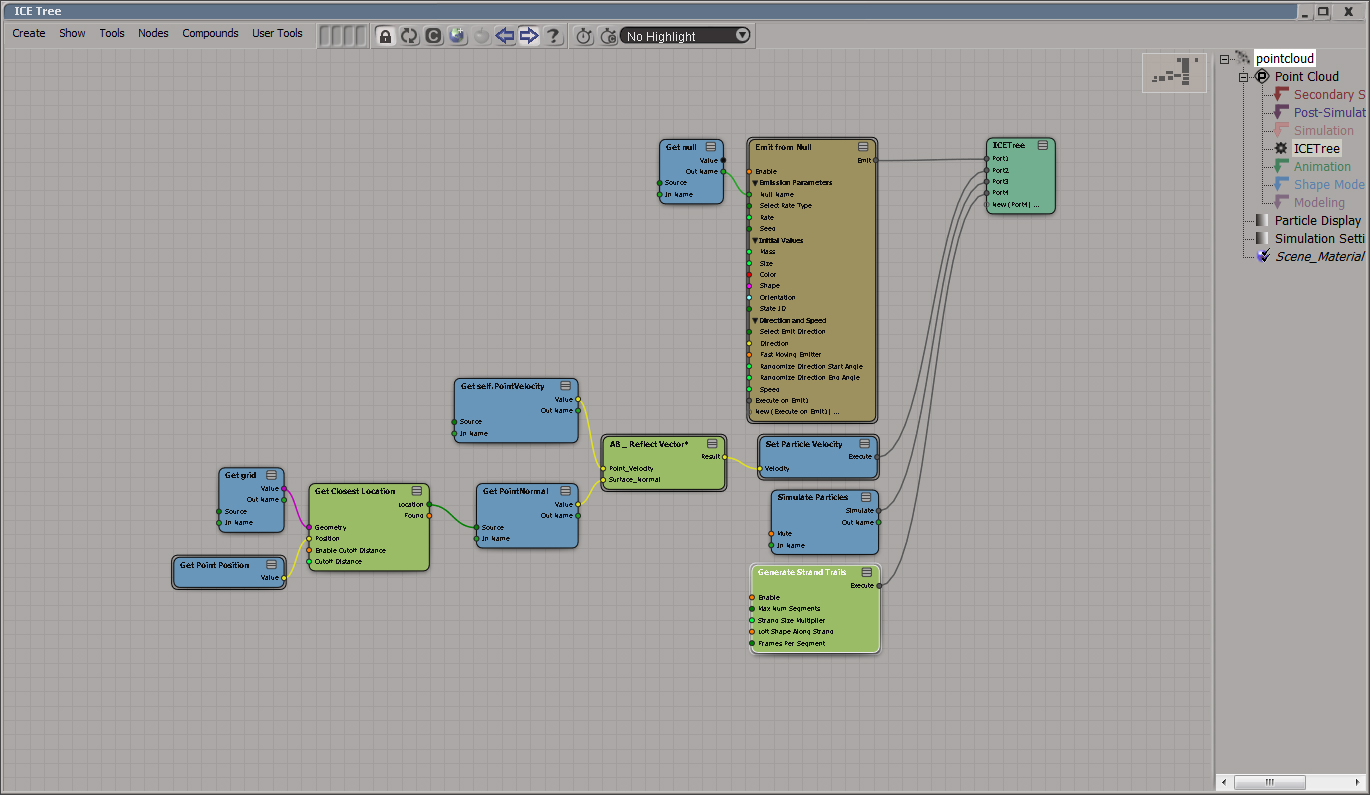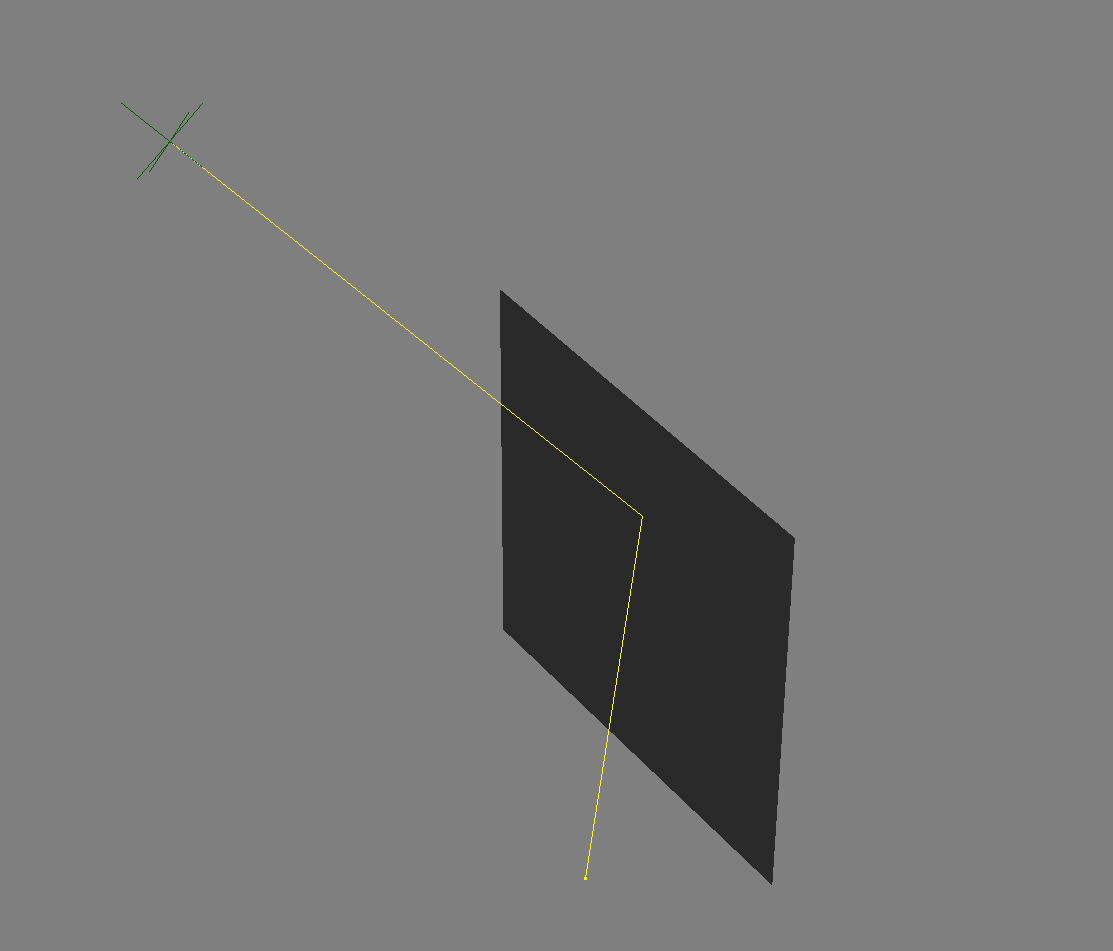# Law of reflection in ICE

A few days we were thinking of, how we could do a proper reflection of of a vector that hits a surface. So we looked up Wikipedia and found the proper explanation and a formula for this case.

As Wikipedia says:“…the direction of incoming light (the incident ray), and the direction of outgoing light reflected (the reflected ray) make the same angle with respect to the surface normal, thus the angle of incidence equals the angle of reflection, and that the incident, normal, and reflected directions are coplanar.”

Good. How can we port this to ICE?Look at the formula from Wikipedia. With some basic notes I was able to rebuild it.AB _ Reflect Vector.zip